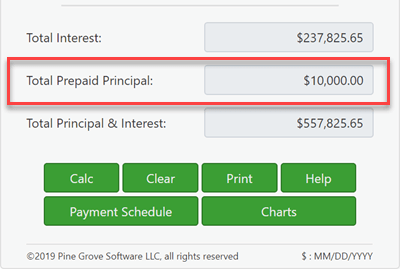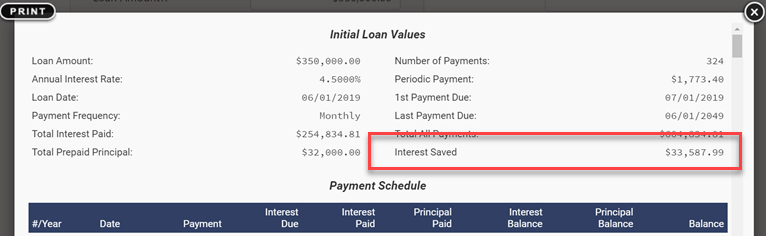# Loan CalculatorCalculate loan payment, term, interest rate, or amount.

advertisement

## How to Use the Loan CalculatorLoan Calculator

Calculating a loan payment amount with this calculator is very easy.

1. Click clear and enter values for:
• Loan Amount
• Number of Payments (term)
• Annual Interest Rate
2. Optionally set the dates.
3. Leave Loan Payment Amount set to 0.
4. Click either "Calc" or "Payment Schedule."

You can leave the other dozen or so options untouched unless you have a specific reason for changing them.

×

### Info...

Original Size
New! Drag & drop your saved files here to load...
File save and open are new beta test features. If you happen to get a different calculated result, do not assume that this calculator is making an error. Most likely, the problem is with the new file load feature. Please check that all settings got loaded as expected.

### Always enter (and reenter) a 0 for the unknown value.

Note - You must enter a zero if you want a value calculated.

Why not design the calculator to recalculate the last unknown?

Because we want the calculator to be able to create a payment schedule using the loan terms you need. This behavior is a feature! After all, there is no such thing as a "correct" loan payment. The payment amount is correct as long as both the lender and debtor agree to it! (If the calculator always recalculated the last unknown, then this feature would not be possible.)

### About the loan origination date (start date) and first payment date.

Important - The first loan payment period is seldom equal to the frequency of other schedule payments. That is, if a loan's payment schedule is monthly, the time from when the loan originates (when the borrower receives the money) until the day the first payment is due will likely not equal one month. The first period will typically be either longer or short than a month.

A longer or shorter first period impacts the interest calculation.

Very few (if any?) online calculators can correctly handle this detail. But if you want accurate interest and payment calculations, you need to be able to independently set the loan origination date and the first payment due date. You can do that on the "Options" tab of this calculator.

Warning - Selecting dates will result in payment amounts as well as interest charges that do not match other calculators.

That's the point!

If you want to match other calculators, then set the "Loan Date" and "First Payment Due" so that the time between them equals one full period as set in "Payment Frequency." Example: If the "Loan Date" is May 15th and the "Payment Frequency" is "Monthly," then the "First Payment Due" should be set to June 15th, that is IF you want a conventional interest calculation.

See "Long Period Options" and "Short Period Options" below for additional details about payment amounts and interest calculations.

Yet keeping it simple - if you only need estimates and not absolute accuracy, you can always leave the dates set as they are when the calculator loads.

## Much More Than a Payment Calculator

advertisement

### The four values you'll need to set:

• Loan Amount - the principal amount borrowed. It does not include interest.
• Number of Payments (term) - the "Payment Frequency" setting impacts the loan's term. For a loan term of five years, if the payment frequency is monthly, you need to enter 60 for the number of payments. (60 months = 5 years)
• Annual Interest Rate - the nominal interest rate. This the quoted interest rate for the loan. (If the lender is quoting anything other than an annual interest rate, you probably should avoid the loan.)
• Payment Amount - the amount that is due on each payment due date.

Set one of the above to 0 if unknown.

How much can I borrow?

1. set the loan amount to "0" (zero)
2. enter the number of payments
3. enter the annual interest rate, and
4. enter the desired or expected payment amount
5. calculate

How long will it take to pay a loan off?

1. enter the loan amount
2. set the number of payments to "0" (zero)
3. enter the annual interest rate, and
4. enter the desired or expected payment amount
5. calculate

What interest rate allows me to pay \$350 a month?

1. enter the loan amount
2. enter the number of payments
3. set the annual interest rate to "0" (zero), and
4. enter \$350 for the payment amount
5. calculate.

### Three loan options you most likely don't need to touch.

• Payment Frequency - set how often payments are scheduled. The calculator supports 11 options, including biweekly (every other week), monthly, and annually. The schedule calculates payment due dates from the first payment due date.
• Compounding - usually, you should set the compounding frequency to be the same as the payment frequency. Doing so results in simple, periodic interest. Setting this option to "Exact/Simple" results in simple, exact day interest.
• Amortization Method - leave this setting set to "normal" unless you have a specific reason for setting it otherwise. For a complete explanation of these options, see Nine Loan Amortization Methods.

### Results - your loan summaryLoan calculator showing lump sum payment made.See the payment schedule for total interest saved.
• Total Interest - assuming the debtor makes the payments as scheduled, this is the interest they will pay over the term of the loan.
• Total Prepaid Principal - this is the total of any extra payments. Note, the total interest saved is reported on the payment schedule.
• Total Principal & Interest - the loan amount plus the total interest paid. Thus the total amount you'll pay for the loan.

### Eleven loan options you may want to tweak.

• Loan Date - the date the money is available. If the loan is for a vehicle or home, it is the loan's closing date.
• First Payment Due - for leases, it may be the same as the loan date. See "About the loan origination date (start date) and first payment date" above.
• Extra Payment Amount - want to make a single extra payment or series of additional payments? Enter the amount here.
• Extra Payments Start - enter the date you want extra payments to start. The date does not have to align with payment due dates. If you pay a loan monthly and payments are due on the first, you may want to make extra payments on the 15th to align with your pay periods.
• Extra Payment Frequency - set how frequently you'll make additional payments. Want to make extra payments annually when you receive a year-end bonus? This calculator will accommodate such a plan.
• Number of Extra Pmts - enter one or any integer value. If you want to make the extra payments until you pay off the loan, enter "U" for "Unknown."
• Days Per Year - 360/365 days per year option. This setting impacts interest calculations when you set compounding frequency to a day based frequency (daily, exact/simple or continuous) or when there are odd days caused by an initial irregular length period.
• Rounding Options - due to payment and interest rounding each pay period (for example, payment or interest might calculate to 345.0457, but a schedule will round the value to 345.05), almost all loan schedules need a final rounding adjustment to bring the balance to "0". A footnote on the payment schedule informs you of the rounding amount.
• Long Period Options (odd day interest) - setting for how interest is shown on the schedule when the initial period is longer than the selected payment frequency.
• Short Period Options - setting for how payments get adjusted when the initial period is shorter than the selected payment frequency.
• Fiscal Year-End - this setting establishes after what month the calculator shows year-end and running totals. This option is to accommodate businesses with fiscal year ends that do not coincide with the calendar year-end.

More details about the settings for odd day and irregular period interest.Amortization schedule shows the interest saved due to extra payments.

advertisement

## 114 Comments on “Loan Calculator”

Join the conversation. Tell me what you think.

advertisement